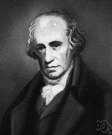# watt

(redirected from Zettawatt)
Also found in: Thesaurus, Medical, Encyclopedia.
Related to Zettawatt: microwatt, Zettabyte

## watt

(wŏt)
n. Abbr. W Electricity
An SI-derived unit of power equal to one joule per second. See Table at measurement.

[After James Watt.]

## Watt

(wɒt)
n
(Biography) James. 1736–1819, Scottish engineer and inventor. His fundamental improvements to the steam engine led to the widespread use of steam power in industry

## watt

(wɒt)
n
(Units) the derived SI unit of power, equal to 1 joule per second; the power dissipated by a current of 1 ampere flowing across a potential difference of 1 volt. 1 watt is equivalent to 1.341 × 10–3 horsepower. Symbol: W
[C19: named after James Watt]

## watt

(wɒt)

n.
the SI unit of power, equivalent to one joule per second and equal to the power in a circuit in which a current of one ampere flows across a potential difference of one volt. Abbr.: W, w
[1882; after J. Watt]

## Watt

(wɒt)

n.
James, 1736–1819, Scottish engineer and inventor.

## watt

(wŏt)
A unit used to measure power, equal to one joule of work per second. In electricity, a watt is equal to the amount of current (in amperes) multiplied by the amount of potential (in volts).

## watt

1. The unit of power: one watt equals one joule per second.
2. (W) A unit of power equal to that available when one joule of energy is expended in one second. 1 W = 1 volt-ampere; 746 W = 1 horsepower (hp). Named after the Scottish engineer James Watt (1736–1819).
ThesaurusAntonymsRelated WordsSynonymsLegend:
 Noun 1watt - a unit of power equal to 1 joule per second; the power dissipated by a current of 1 ampere flowing across a resistance of 1 ohmWpower unit - a measure of electric powermilliwatt - a unit of power equal to one thousandth of a wattkilowatt, kW - a unit of power equal to 1000 wattsH.P., horsepower, HP - a unit of power equal to 746 watts 2Watt - Scottish engineer and inventor whose improvements in the steam engine led to its wide use in industry (1736-1819)James Watt
Translations
واط: وَحْدَة القُدْرَه الكَهْرُبائِيَّه
ват
watt
watt
watti
watt
vatt
ワット
vats
wat
watt
vatwatt
watt

[wɒt] N

## watt

[ˈwɒt] nwatt m
a 100-watt light-bulb → une ampoule de 100 watts

nWatt nt

## watt

[wɒt] nwatt m inv

## watt

(wot) noun
(abbreviated to W when written) a unit of power, especially of heat or light.
Site: Follow: Share:
Open / Close